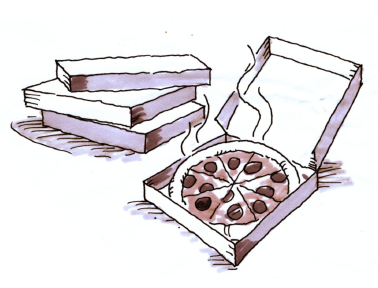### Home > CC2 > Chapter 6 > Lesson 6.2.4 > Problem6-97

6-97.

For the end-of-year party, Mt. Rose Middle School ordered $112$ pizzas. There were eight fewer veggie pizzas than there were pepperoni pizzas. There were three times as many combo pizzas as pepperoni pizzas. Use the 5-D Process to define a variable and write an equation for this situation. Then determine how many of each kind of pizza were ordered.Let $p=\text{# of pepperoni pizzas}$. Then $3p=\text{# of combo pizzas}$. Write an expression to describe the number of veggie pizzas.

The equation should be... $(\text{# of pepperoni pizzas})+(\text{# of}\ \dots)+()=112.$

Be sure you know how to get these answers.

$p+(p-8)+3p=112$

$16$ veggie, $24$ pepperoni, and $72$ combo pizzas.